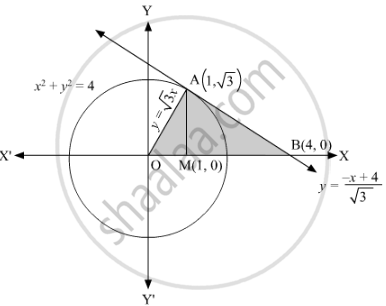# Using integration find the area of the triangle formed by positive x-axis and tangent and normal of the circle - Mathematics

Using integration find the area of the triangle formed by positive x-axis and tangent and normal of the circle

x^2+y^2=4 at (1, sqrt3)

#### Solution

The given equation of the circle is x2+y2=4.

The equation of the normal to the circle at (1,√3) is same as the line joining the points (1,√3) and (0, 0), which is given by

(y−sqrt3)/x−1=(sqrt3−0)/(1−0)

(y−sqrt3)/x−1=sqrt3

⇒y−sqrt3=sqrt3x−sqrt3

⇒y=sqrt3x                    .....(1)

So, the slope of normal is sqrt3.

We know that the product of the slopes of the normal and the tangent is 1

Therefore, the slope of tangent is −1/sqrt3

Now, the equation of the tangent to the circle at (1,√3) is given by

(y−sqrt3)/x−1=-1/sqrt3

⇒sqrt3y−3=−x+1

y=−(x+4)/sqrt3          .....(2)

Putting y = 0 in (2), we get x = 4.

Thus, ABC is the triangle formed by the positive x-axis and tangent and normal to the given circle at (1,sqrt3)

.Now,

Area of ∆AOB = Area of ∆AOM + Area of ∆AMB

=int_0^1ydx+int_1^4y dx

=int_0^1sqrt3xdx+int_1^4((-x+4)/sqrt3)dx

=[(sqrt3x^2)/2]_0^1+int_1^4-x/sqrt3dx+int_1^44/sqrt3dx

=(sqrt3/2-0)-[x^2/(2sqrt3)]_1^4+[4/sqrt3x]_1^4

=sqrt3/2-16/(2sqrt3)+1/(2sqrt3)+16/sqrt3-4/sqrt3

=sqrt3/2+(3sqrt3)/2

=2sqrt3

Thus, the area of the triangle so formed is 2sqrt3 square units.

Concept: Area Under Simple Curves
Is there an error in this question or solution?
Share